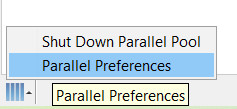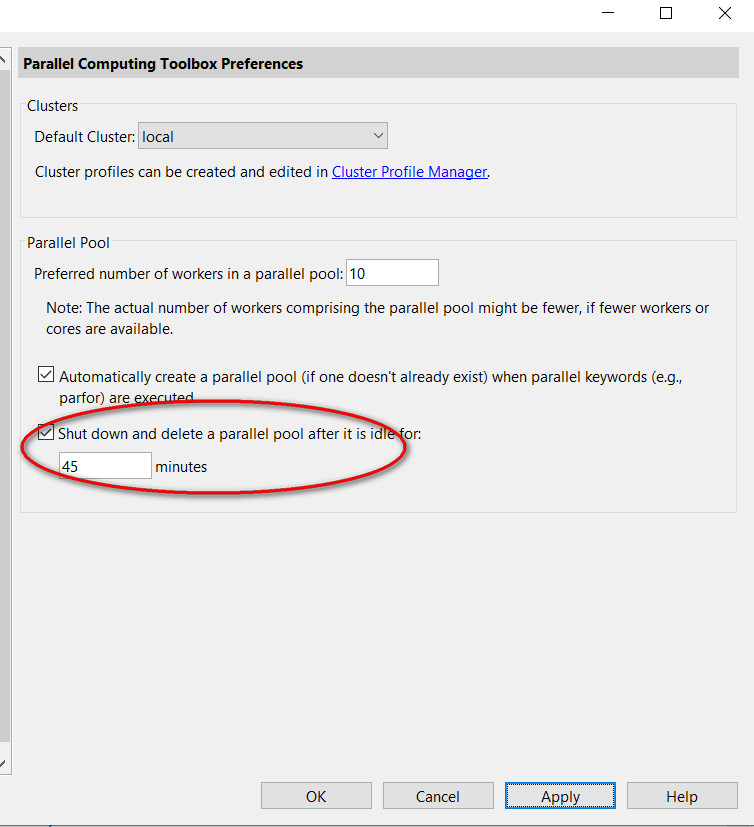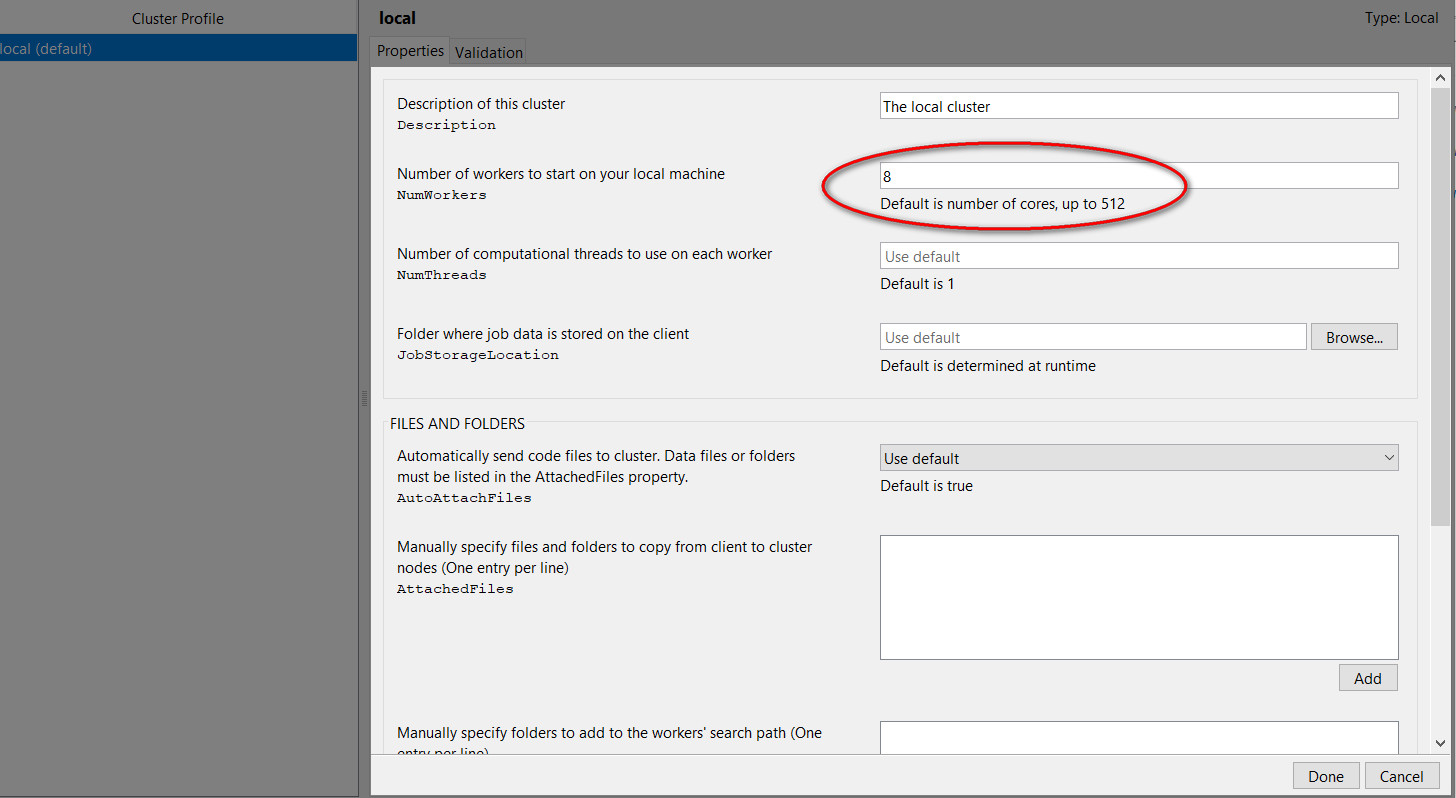# How to use parallel pools in MATLAB with real examples

Last updated on：2 years ago

The parallel pool is a tool that allows MATLAB to make full use of your CPU, which means it can save time to run your codes. I’ll teach you how to use this tool by given some engineering examples.

When you need to call the same function with different parameters or directly call several different functions, you may ask, “Why my computer cannot fully utilize CPU and memory to enhance computational capabilities?” At this time, the Parallel Pool appears. As the name implies, you can run your program in parallel, such as using two sets of parameters to call the same function and run them at the same time.

# Result

I give you some results first. The following results compare two sets of input parameters calling the same function. With the help of parallel pools, my programme is 12 seconds faster than the ordinary one.

Got result with index: 1.
Got result with index: 2.
Elapsed time is 131.705283 seconds.
Elapsed time is 143.168543 seconds.

For sets of input parameters (I don’t know why the ordinary one is so slow):

Elapsed time is 519.250776 seconds.
Elapsed time is 1362.264277 seconds0.

# Parallel Pool Configuration# parfeval() function

According to the official document, it is defined as an execute function asynchronously on parallel pool worker. In other words, the function is executed in the workers of the parallel pool at different times. I think it is to emphasize that the parallel pool also has limited space.

Syntax

F = parfeval(p,fcn,numout,in1,in2,...)
F = parfeval(fcn,numout,in1,in2,...)

p(pool) are other configurations of the parallel pool, such as the number of workers; fcn is the name of the input function; numout is the number of function output parameters (it can be 0); in1, in2, in3 are input parameters (the input parameters are totally the same when calling the function fcn).

# Application

## Official Example

The official code is to run the magic(N) function 10 times in parallel, idx is the input parameter N. Thus, there are ten sets of input data, from 1 to 10. Parfeval function is used here. FevalFuture is established in the first loop, and the input data is bound to the function name; the result will be calculated from [completedIdx, value] = fetchNext(f) in the second loop.

num = 10; % You can set the num with different values to test
f(1:num) = parallel.FevalFuture;
for idx = 1:num
f(idx) = parfeval(@magic,1,idx);
end

magicResults = cell(1,num);
for idx = 1:num
[completedIdx,value] = fetchNext(f);
magicResults{completedIdx} = value;
fprintf('Got result with index: %d.\n', completedIdx);
end

The equal function is shown as follow.

magicResults = cell(1,num);
for idx = 1:num
magicResults{idx} = magic(idx);
end

Add tic toc to count the time. ( The first line is the time using parallel pool, the other is the time without utilizing parallel pool)

num = 10;

Elapsed time is 0.264016 seconds.
Elapsed time is 0.000820 seconds.

num = 1000;

Elapsed time is 11.995380 seconds.
Elapsed time is 3.562912 seconds.

num = 1500;

Elapsed time is 28.925654 seconds.
Elapsed time is 12.127613 seconds.

It can be seen that the time ratio is getting smaller and smaller, but this program is still relatively faster without parallelism. Therefore, parallelism should be used with caution.

## Project Example

Declaration of function：

function kuangXiaoHu(FOI,SepParam)

I simplify my batch programme, and just apply 2 sets of input parameters to the function.

matlab
geoparam.shape = 2;
FOI = 'ellipsoid'; % field of interest
%% parallel
tic
idex = 0;
f1(1:2) = parallel.FevalFuture;
for theta = [30, 60]
SepParam.theta = theta;
idex = idex + 1;
f1(idex) = parfeval(@kuangXiaoHu,0,FOI,SepParam);
end
%%%%%% solve
num = length(theta);
for idx = 1:num
completedIdx = fetchNext(f(idx));
magicResults{completedIdx} = value;
fprintf('Got result with index: %d.\n', completedIdx);
end
toc
%% unparallel
tic
for theta = [30 60]
sepparam.theta = theta;
kuangxiaohu(FOI,SepParam);
end
toc

You can see the result in the former. It save 10 + seconds in this example, and it could be much faster by adding more sets of input data.

# Other Method

You can open another MATLAB to run your programme, the result would be the same as you use parallel pool. But it occupies much space.